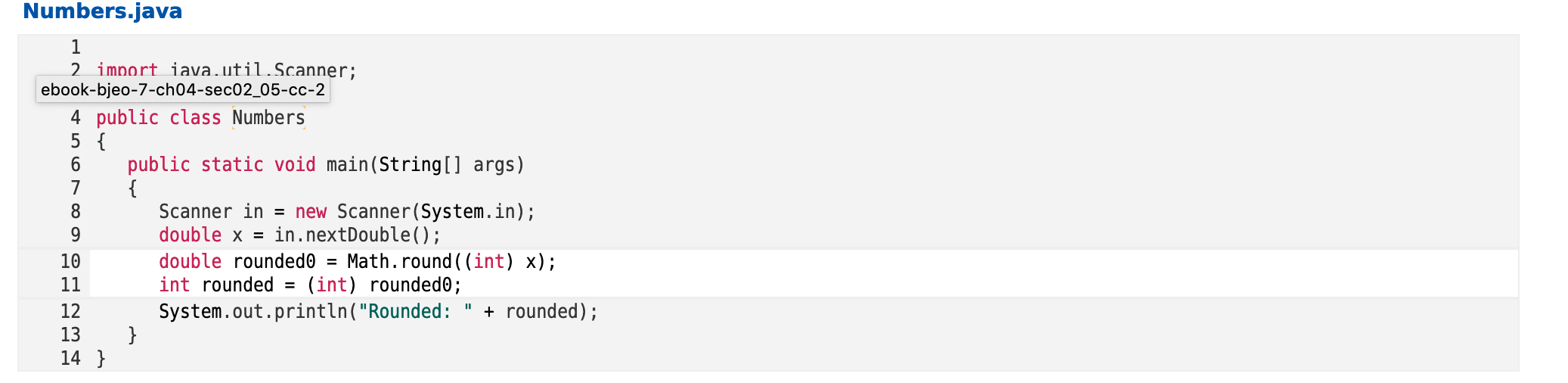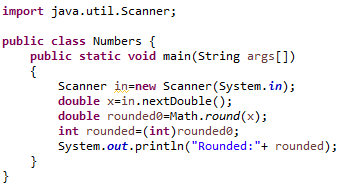# Numbers.java2 import iava.util.Scanner;ebook-bjeo-7-ch04-sec02_05-cc-24 public class Numbers5 {public static void main(String[] args){Scanner in = new Scanner(System.in);double x = in.nextDouble();double rounded0 = Math.round ( (int) x);9.10(int) rounded@;int rounded11+ rounded);System.out.println("Rounded:}121314 }

Question
8 views

In Ruritania, fractional numbers are rounded up to the next integer if the fractional part is at least 0.25. However, if they are negative, they are rounded down to the next lower integer if the fractional part is more than 0.75. For example,

2.5 → 3
2.25 → 3
2.24 → 2
-2.3 → -2
-2.76 → -3
-3.25 → -3

Complete the following program to print out the integer, using Ruritanian rounding.

No loops or if statements.help_outlineImage TranscriptioncloseNumbers.java 2 import iava.util.Scanner; ebook-bjeo-7-ch04-sec02_05-cc-2 4 public class Numbers 5 { public static void main(String[] args) { Scanner in = new Scanner(System.in); double x = in.nextDouble(); double rounded0 = Math.round ( (int) x); 9. 10 (int) rounded@; int rounded 11 + rounded); System.out.println("Rounded: } 12 13 14 } fullscreen
check_circle

Step 1: Java Source Code

import java.util.Scanner;

public class Numbers {

public static void main(String args[])

{

Scanner in=new Scanner(System.in);

double x=in.nextDouble();

double rounded0=Math.round(x);

int rounded=(int)rounded0;

System.out.println("Rounded:"+ rounded);

}

}

Step 2: Screenshot of the Code...

### Want to see the full answer?

See Solution

#### Want to see this answer and more?

Solutions are written by subject experts who are available 24/7. Questions are typically answered within 1 hour.*

See Solution
*Response times may vary by subject and question.
Tagged in

### Computer Science